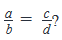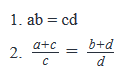If a, b, c and d are positive real numbers, i...
If a, b, c and d are positive real numbers, is• a)
Statement (1) ALONE is sufficient, but statement (2) alone is not sufficient to answer the question asked.
• b)
Statement (2) ALONE is sufficient, but statement (1) alone is not sufficient to answer the question asked.
• c)
BOTH statements (1) and (2) TOGETHER are sufficient to answer the question asked, but NEITHER statement ALONE is sufficient to answer the question asked.
• d)
EACH statement ALONE is sufficient to answer the question asked.
• e)
Statements (1) and (2) TOGETHER are NOT sufficient to answer the question asked, and additional data specific to the problem are needed.
Correct answer is option 'B'. Can you explain this answer?

### Related TestAparna Tammana Sep 02, 2022
Related If a, b, c and d are positive real numbers, is a)Statement (1) ALONE is sufficient, but statement (2) alone is not sufficient to answer the question asked.b)Statement (2) ALONE is sufficient, but statement (1) alone is not sufficient to answer the question asked.c)BOTH statements (1) and (2) TOGETHER are sufficient to answer the question asked, but NEITHER statement ALONE is sufficient to answer the question asked.d)EACH statement ALONE is sufficient to answer the question asked.e)Statements (1) and (2) TOGETHER are NOT sufficient to answer the question asked, and additional data specific to the problem are needed.Correct answer is option 'B'. Can you explain this answer?
2.(a+c)/c = (b+d)/d
a/c + 1 = b/d + 1
a/c = b/d
a/b = c/d
2 is sufficient to answer
1.ab=cd with this we can't say if a/b = c/d or not
therefore 2 alone is sufficient to answer

 1 Crore+ students have signed up on EduRev. Have you?